### IMO Shortlist 2011 problem N6

Kvaliteta:
Avg: 0,0
Težina:
Avg: 7,5
Let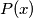$P(x)$ and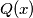$Q(x)$ be two polynomials with integer coefficients, such that no nonconstant polynomial with rational coefficients divides both$P(x)$ and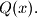$Q(x).$ Suppose that for every positive integer$n$ the integers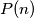$P(n)$ and$Q(n)$ are positive, and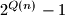$2^{Q(n)}-1$ divides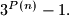$3^{P(n)}-1.$ Prove that$Q(x)$ is a constant polynomial.

Proposed by Oleksiy Klurman, Ukraine
Izvor: Međunarodna matematička olimpijada, shortlist 2011